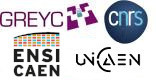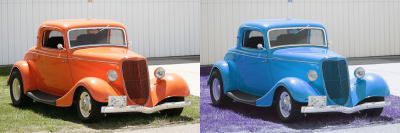G'MIC - GREYC's Magic for Image Computing: A Full-Featured Open-Source Framework for Image Processing# A Full-Featured Open-Source Framework for Image Processing

## Latest stable version: 3.1.4

Tutorial

mix_rgbTaking out the old Ford coupe for a 190° spin around the white vector.

This command multiplies each pixel in the selected images by a 3x3 matrix composed of the nine given arguments. That is:

gmic                 \
-sample car       \
-mix_rgb <a11>,<a12>,<a13>,<a21>,<a22>,<a23>,<a31>,<a32>,<a33> \

uses the nine arguments to assemble a matrix that then multiplies each pixel of the selected image:The matrix generally scales and rotates pixels, which are taken as colorspace vectors. The resulting image almost always requires normalization before use in paint programs. See norm and orientation for more information on the treatment of pixels as color space vectors.

This command is rarely used with manually written arguments; composing color space rotation matrices on the command line is a distinctly non-intuitive approach to adjusting image hues. Consider adjust_colors for a more intuitive command that adjusts an image's hue, saturation, value and gamma.

More typically, mix_rgb 's arguments are generated in scripts. This example harnesses the math expression parser to generate a rotation matrix about the white axis, twisting the coupe's original orange to somewhere between cyan and blue, the grass becoming purple. The right hand Ford coupe, above, is the result.

gmic                       \
-sample car,200         \
-mix_rgb. '{whitev=vector3(1/sqrt(3));rot(whitev,190°)}' \

In a similar - perhaps frivolous - vein, one may pick the color of a particular pixel and harness that as the axis of rotation around which all other colors spin:

 fixedblue fixedbeach constant sky constant beach sand
gmic                                       \
-sample cliff,300                       \
-repeat 359                             \
+fill "begin(                     \
vec=I(<x>,<y>);     \
vec=vec/norm(vec)   \
);                    \
rot(vec,($>+1)°)*I" \ -done \ -normalize 0,255 \ -output img/fixedblue.mp4,24 \ The left rotates around a blue sky vector sampled at <x>,<y> = [110,110]; the right rotates around a buff color vector sampled from the beach at <x>,<y> = [106,177]. As the colors of these pixels serve as axes of rotation they are least changed by the rotation. The sky changes little on the left, always more-or-less blue; the beach on the right remains more-or-less sandy. Command Reference$ gmic -h mix_rgb

mix_rgb:
a11,a12,a13,a21,a22,a23,a31,a32,a33

Apply 3x3 specified matrix to RGB colors of selected images.

Default values: 'a11=1', 'a12=a13=a21=0', 'a22=1', 'a23=a31=a32=0' and 'a33=1'.

Example:
[#1] image.jpg +mix_rgb 0,1,0,1,0,0,0,0,1

Tutorial: https://gmic.eu/tutorial/mix_rgb
G'MIC - GREYC's Magic for Image Computing: A Full-Featured Open-Source Framework for Image Processing